# Numerical Simulation of Free Convection in a Three-Dimensional Enclosure Full of Nanofluid with the Existence a Magnetic Field

Numerical Simulation of Free Convection in a Three-Dimensional Enclosure Full of Nanofluid with the Existence a Magnetic Field
LGEMS, Ibn Zohr University, ENSA, Agadir BP 1136, Morocco

Corresponding Author Email:
saidbouchta.doc@gmail.com
Page:
405-411
|
DOI:
https://doi.org/10.18280/ejee.220602
Received:
3 May 2020
|
Accepted:
11 November 2020
|
Published:
31 December 2020
| Citation

OPEN ACCESS

Abstract:

A numerical analysis was performed to study the influence of a magnetic field in free convection in a cube full with nanofluid. To solve the equation, we appeal to finite volume method. The SIMPLEC algorithm is used for pressure-velocity coupling. All walls are adiabatic, except for the left and right walls that are heated differently. The effects of the Rayleigh and Hartmann numbers, as well as the volume fraction of nanometric particles were studied. Results are conveyed in the form of isotherms, streamlines, velocity curves and Nusselt numbers. It has been shown that as the percentage of nanoparticles increases and the number of Rayleigh increases, heat transfer improves. Hartman number has considerable influence on hydrodynamic and thermal field.

Keywords:

three-dimensional, nanofluid, magnetic field, convection, finite volume method, SIMPLEC, Hartmann number, numerical simulation

1. Introduction

Conventional liquids used in free convection, for example water, ethylene glycol or oil have a limited thermal conductivity, which led to replacing them with a new fluid named by physicists, nanofluid. Nanofluids are colloidal solutions composed of nanoparticles suspended in a base liquid, invented by Choi and Eastman . Nanofluids have an important thermal conductivity and show significant progress in convection .

Nanofluids can be used everywhere where large heat flows must be evacuated: heat exchangers, cooling of electrical and electronic components, radars, automobile ...

Many researchers are concentrating their research on nanofluids. Khanafer et al.  examined Numerically the free convection of nanofluids. Their results showed that in any Grashof number, nanoparticles added to the base fluid significantly increase the heat transfer rate. Abu-Nada and Oztop  have shown that heat transfer is very crucial with increasing of nanoparticles. Oztop and Abu-Nada  carried out two dimensions investigation of the free convection of diverse nanofluids in partially heated enclosure, they showed that the genre of nanoparticles was a key essential in improving heat transfer. They obtained the best results using copper nanoparticles.

Other investigators have investigated several nanofluid-filled geometries [6-8], different thermal boundary conditions, [9-11], and different internal shapes [12, 13] for improvement and intensification of heat exchange.

Free convection in 3D cavities fulled of nanofluid has been addressed by few researchers, such as Ravnik et al.  analyzed free convection in a cube fulled with nanofluids using the Border Element Method (BEM). El Moutaouakil et al.  was investigates the effect of thermal radiation on natural convection of various nanofluids in a partially heated cubical cavity. They studied the effect on the dynamic and thermal fields as well as the heat transfer of several parameters, the volume fraction, the radiation parameter, the aspect ratio of the heated elements, and the angle of inclination of these elements (0°, 45° and 90°). Selimefendigil and Oztop  treated heat transfer by convection of carbon nanotube/water nanofluids in 3D cavity separated by a conductive partition and having an internal rotating adiabatic circular cylinder. They analyzed the influence on the heat transfer of various parameters, Richardson number, the angular rotation velocity of the inner cylinder, the thickness and the solid fraction φ.

Atashafrooz  studied numerically in 3D, the circulation of nanofluid in an inclined step. He showed that the nanoparticle volume fraction acts more on the temperature distribution than on the velocity distribution, and increases the coefficient of friction, temperature and Nusselt number.

A three-dimensional simulation of the mesoscopic scale of natural magnetohydrodynamic convection using the Lattice Boltzmann method has been examined by Sajjadi et al. . The findings show that increasing the Hartmann number considerably weakens the heat transfer. In addition, the influence of the Ha increases with the improvement of the Grashof number, such as the reduction of the average number of Nusselt. Zhou et al.  Also used the method Boltzmann, they established a three-dimensional model to analyzing the flow dynamics and heat transfer of mixed convection of Al2O3 fluid in a three-dimensional cavity in the presence of a magnetic field. Different parameters were taken into consideration in this study of the flow of nanofluids and heat transfer such as Rayleigh, Hartmann and Richardson numbers, nanoparticle volume fraction. The conclusions show that a low Ra, the addition of particles to water can improve heat transfer effect. However, at high Rayleigh numbers, this improvement in heat transfer may weaken or even reverse. In addition, the conclusions show that the applied outside magnetic field has the influence of disappearing the convection state of heat transfer in the enclosure.

Al-Sayegh  has numerically analyzed the free convection of a carbon nanotube (CNT)-nanofluid within an open trapezoidal enclosure under action of an inclination of the outside magnetic field by the finite volume method. The analysis took into account several factors, note the Rayleigh and Hartmann numbers, the solid fraction, and the angle of inclination of the magnetic force. It has been found that, for high values of Ra, the heat transfer rate decreases when Hartmann number is increased, while this heat transfer rate improves with increasing volume fraction of the nanoparticles regardless of the angle of inclination of magnetic field.

A numerical analysis of the natural convection of CNT nanofluids, based on finite volumes is carried by Al-Rashed et al. , they analyzed the influence of adequate factors, such as the Rayleigh number, the nanoparticles volume fraction, angle of inclination of the outer magnetic field and the Hartman number. They show that heat transfer improves with the addition of CNT particles and Rayleigh number. When Ha goes from 50 to 100, magnetic repulsion inhibits heat transfer by 50%.

The objective of this work is to study the flow of a nanofluid (Cu / water) passing through a cube subjected to a magnetic field, as well as to quantify convective exchanges. The effects of nanofluid, the magnetic field as well as the influence of the nanoparticle concentration on the thermal performance of the cavity, are analyzed in this study.

2. Mathematical Modelling

The study geometry (Figure 1) is a three-dimensional enclosure which is full with and Copper nanoparticles with Pr=6.2 and heated differently (Th > Tc). The other walls are assumed adiabatic. It is subjected to the influence of a constant and uniform outer magnetic field in the horizontal direction.

For the modeling of the physical problem described in Figure 1, we will adopt the following simplifying hypotheses: the flow is laminar, and the fluid is incompressible and Newtonian. Apart from the density that evolves according to the Boussinesq approximation of Bejan , all thermophysical properties (at 300 K) listed in Table 1, are considered constant.

## 1.png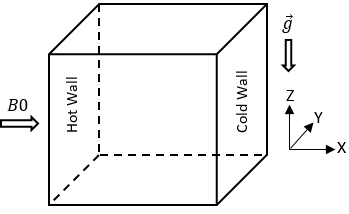Figure 1. Cavity geometry

Table 1. Thermophysical properties of nanofluid with T=300K 

 Thermophysical properties Water Cu cp (J kg-1 K-1) 4179 385 ρ (kg m-3) 997.1 8933 k (W m-1K-1) 0.613 401 β (K−1) 2.761×10-4 1.67×10-5 α (m2 s-1) 1.47×10-7 - σ(μs/cm) 0.05 5.96×107

Our system of equations, in steady state, of conservation of mass, conservation of momentum and energy that governs the configuration studied in its dimensionless form is given by:

$\frac{\partial U}{\partial X}+\frac{\partial V}{\partial Y}+\frac{\partial W}{\partial Z}=0$           (1)

$U\left(\frac{\partial U}{\partial X}\right)+V\left(\frac{\partial U}{\partial Y}\right)+W\left(\frac{\partial U}{\partial Z}\right)=-\frac{\partial P}{\partial X}+\frac{\mu_{n f}}{\rho_{n f} \alpha_{f}}\left(\frac{\partial^{2} U}{\partial X^{2}}+\frac{\partial^{2} U}{\partial Y^{2}}+\frac{\partial^{2} U}{\partial Z^{2}}\right)$            (2)

\begin{aligned} U\left(\frac{\partial V}{\partial X}\right)+V\left(\frac{\partial V}{\partial Y}\right)+W\left(\frac{\partial V}{\partial z}\right) =&-\frac{\partial P}{\partial Y}+\frac{\mu_{n f}}{\rho_{n f} \alpha_{f}}\left(\frac{\partial^{2} V}{\partial X^{2}}+\frac{\partial^{2} V}{\partial Y^{2}}+\frac{\partial^{2} V}{\partial Z^{2}}\right)-H a^{2} P r V \end{aligned}             (3)

$U\left(\frac{\partial W}{\partial X}\right)+V\left(\frac{\partial W}{\partial Y}\right)+W\left(\frac{\partial W}{\partial Z}\right)=-\frac{\partial P}{\partial Z}+\frac{\mu_{n f}}{\rho_{n f} \alpha_{f}}\left(\frac{\partial^{2} W}{\partial X^{2}}+\frac{\partial^{2} W}{\partial Y^{2}}+\frac{\partial^{2} W}{\partial Z^{2}}\right)+\frac{(\rho \beta)_{n f}}{\rho_{n f} \beta_{f}} R a \operatorname{Pr} \theta-H a^{2} \operatorname{Pr} W$                (4)

\begin{aligned} U\left(\frac{\partial \theta}{\partial X}\right)+V\left(\frac{\partial \theta}{\partial Y}\right) &+W\left(\frac{\partial \theta}{\partial Z}\right)=\frac{\alpha_{n f}}{\alpha_{f}}\left(\frac{\partial^{2} \theta}{\partial X^{2}}+\frac{\partial^{2} \theta}{\partial Y^{2}}+\frac{\partial^{2} \theta}{\partial Z^{2}}\right) \end{aligned}                (5)

The dimensionless parameters of the previous equations are:

$X=\frac{x}{H}, Y=\frac{y}{H}, Z=\frac{z}{H^{\prime}}, U=\frac{u H}{\alpha_{f}}, V=\frac{v H}{\alpha_{f}} W=\frac{w H}{\alpha_{f}},P=\frac{p H^{2}}{\rho_{n f} \alpha_{f}^{2}}, \theta=\frac{T-T_{c}}{T_{h}-T_{c}}$                  (6)

$R a=\frac{g \beta_{f}(T h-T c) H^{3}}{\alpha_{f} v_{f}}, P r=\frac{v_{f}}{\alpha_{f}}, H a=B_{0} L \sqrt{\frac{\sigma_{n f}}{\rho_{n f} v}}$               (7)

Table 2 shows the thermophysical properties of nanofluid (water-Cu).

Table 2. Thermophysical properties of a nanofluid

 Properties Nanofluid  Density $\rho_{n f}=\left(1-\varphi_{p}\right) \rho_{f}+\varphi \rho_{p}$ Heat capacity $\left(\rho c_{p}\right)_{n f}=\left(1-\varphi_{p}\right)\left(\rho c_{p}\right)_{f}+\varphi\left(\rho c_{p}\right)_{p}$ Thermal expansion coefficient $(\rho \beta)_{n f}=\left(1-\varphi_{p}\right)(\rho \beta)_{f}+\varphi(\rho \beta)_{p}$ Viscosity $\mu_{n f}=\frac{\mu_{f}}{\left(1-\varphi_{p}\right)^{2.5}}$ Thermal conductivity $\frac{k_{n f}}{k_{f}}=\frac{k_{p}+2 k_{f}-2 \varphi_{p}\left(k_{f}-k_{p}\right)}{k_{p}+2 k_{f}+\varphi_{p}\left(k_{f}-k_{p}\right)}$ Thermal diffusivity $\alpha_{n f}=\frac{k_{n f}}{\left(\rho c_{p}\right)_{n f}}$ Electrical conductivity $\sigma_{n f}=\left(1-\varphi_{p}\right) \sigma_{f}+\varphi \sigma_{p}$

The Eqns. (1)-(5) obtained are solved with the following boundary conditions:

U=V=W=0             on all the walls        (8)

θ=0.5                      with X=0              (9)

θ=-0.5                   with X= 1                     (10)

∂θ⁄∂n=0             for the others                   (11)

where, n denotes to the normal direction to wall.

The calculation of the average Nusselt number of is based on the following relation:

$N u_{a v g}=\int_{0}^{1} \int_{0}^{1}-\frac{k_{n f}}{k_{f}}\left(\frac{\partial \theta}{\partial X}\right)_{X=0} d Z d Y$             (12)

3. Numerical Methode

We use the finite volume method to discretize the equations of the mathematical model. The obtained equations are solved by the usual iterative method TDMA . The iterative process takes into account the pressure correction by implementing the SIMPLEC algorithm , and reaches convergence when the variation of the dependent variables (U, V, W, P or θ) is no longer significant. A test for stopping the iterative process at convergence is established at each stretch according to the following criterion:

$\sum_{i, j, k=1}^{\text {imax, jmax,kmax }}\quad \frac{\left|\quad\emptyset_{i, j, k}^{n+1}\quad-\quad\emptyset_{i, j, k}^{n}\quad\right|}{\left|\quad\emptyset_{i, j, k}^{n}\quad\right|} \leq 10^{-4}$                   (13)

where, $\emptyset$ is one of the field variables (U, V, W, T, P) and i, j and k the grid positions. n represents the time step number.

To verify the numerical program, the results obtained are compared with the results already available in the literature. First comparison is a three-dimensional numerical simulation of free convection of air and water (Pr = 0.71) at Rayleigh number values ranging from 103 to 106. Table 3 shows comparisons between our results and those of literature. After consultation, we see that our numerical results are in good coherence with those of reference.

Table 3. Comparison our Nusselt number values with 

 Ra Air Water Ravnik et al.  Present Ravnik et al.  Present 103 1,071 1,0607 1,071 1.0639 104 2,0564 2,0464 2,078 2.0583 105 4,3432 4,2979 4,51 4.5237 106 8,6792 8,6461 9,032 9.3897

## 2.png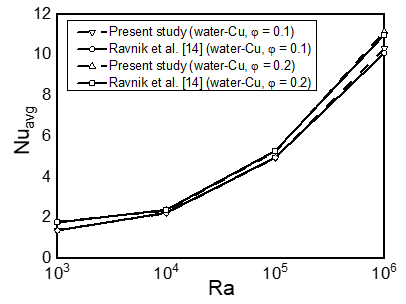Figure 2. Test of our results of average Nusselt number of with results of Ravnik et al.  for Cu-water nanofluid

The second test is still free convection in a three-dimensional cavity but with a Cu-water nanofluid. Our results are compared with those of Ravnik et al.  Figure 2 shows that there is a very good agreement between the average number of Nusselt obtained by this simulation and that obtained by Ravnik et al. .

4. Results

A numerical study was carried by a finite volume method to study the free convection heat transfer and the fluid flow in a cubic cavity exposed to a magnetic field along the x axis, using Cu-water nanofluid. Isotherms, streamlines, velocity profile and variation of average Nusselt number are exposed for different values of Ra (103...107, Ha (0…200), and φ (0…0.05).

Figures 3 and 4 show effect of magnetic field on temperature distribution and fluid flow in cubic enclosure for two values of Ra, φ and Ha.

At Ra=105, as shown in Figure 3, the circulating ﬂows entrained by the buoyancy force in the enclosure are horizontal. Hartman number increases the cells are elongated vertically and tighten upper streamlines. When the Ra is increased from 105 to 107, the strength of the circulating flows also increases, and decreases when the Ha increases from 0 to 200. It should be noted that for a high Ha, the velocity field is suppressed considerably and the conduction is dominant. As Rayleigh number increases Ra=107, as shown in Figure 3, the convection-dominated regime is strengthened, and still remains despite the Hartman number increase. Due to the delaying influence of the Lorenz force generated by the magnetic field imposed on the enclosure, the intensity of the convection force has decreased considerably. The maximum stream function values indicate the reverse effect of the magnetic field.

In Figure 4, at a Rayleigh number 105, for low value of Hartmann number, convection is still dominant, but as Ha increases, the horizontal isotherms transformed parallel and get more and more to the conduction distribution model. For Ra=107, buoyancy force becomes greater and velocity increases, the isotherms also deform since we are in the convection mode which increases the velocity of the flow. We clearly see in Figure 4 that for Rayleigh number 107, the convection regime remains dominant even for a high Hartmann number.

The results of the pure fluid (water, φ=0) are also presented in the same Figures 3 and 4 to make comparisons and highlight the effect of the addition of nanoparticles on the dynamic and thermal field. From the values of the current function shown in Figure 3, the flow of pure fluid is stronger than that of nanofluid in the presence of the magnetic field (Ha>0) while in the absence of the magnetic field (Ha=0), the trend is reversed and the flow of the nanofluid is more intense than that of the pure fluid. This behavior is due to viscosity and Archimedes' strength which depends on density, and both of these factors are important for nanofluids.

Figure 5 shows velocity distribution U at Y=X=0.5 and φ=5% for Ra=105 and Ra=107 as function of Z for two values of Ha. We see the significant decrease in velocity due to the Lorenz force effect when the Hartmann number increases. Whatever value of Ha, the velocity increases from zero near the side wall to a maximum value located in the viscous undercoat. In the heart of the cube, the velocity profile becomes more and more linear for high Hartmann number. The influence of the magnetic field is weaker for Ra=105 than Ra=107.

## 3a.png## 3b.png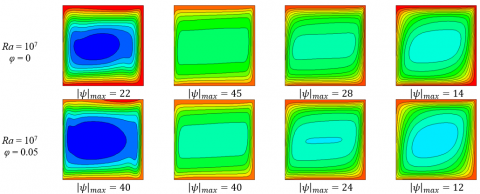Figure 3. Streamlines for different values of Ra, Ha and φ

## 4a.png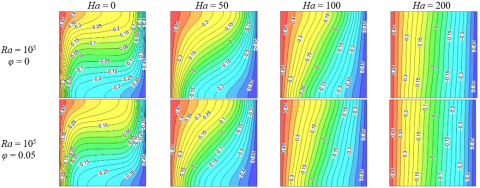## 4b.png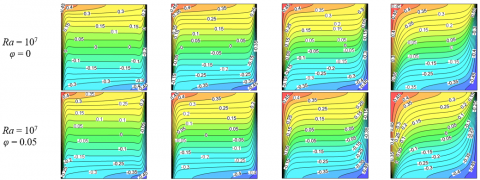Figure 4. Isothermal lines for different values of Ra, Ha and φ

## 5.pngFigure 5. Variation of velocity U at Y = X = 0.5 and φ = 5% with Hartmann number for (a) Ra = 105, (b) Ra =107

## 6a.png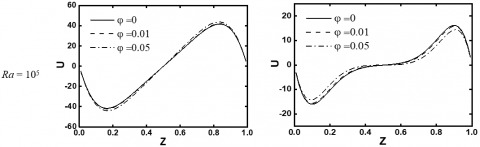## 6b.pngFigure 6. Variation of velocity U at X = Y = 0.5 as a function of φ at different Ra and Ha

## 7.png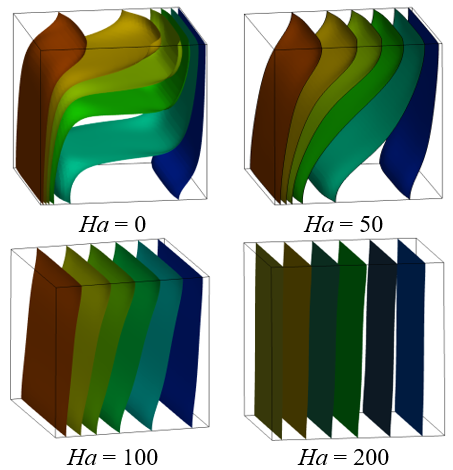Figure 7. Isosurfaces of temperature for Ra = 105 and φ=5%

Figure 6 displays the effect of the increase in nanoparticles on the flow field. At the Rayleigh number 105 and with a relatively large Hartmann number (Ha = 50), the effect of nanoparticles on the intensity of the circulating flow is more marked. Contrarily, at Ra = 107 and Ha = 50, the effect of the addition of nanoparticles on the intensity of the circulating flow is negligible.

Figure 7 shows the temperature isosurfaces for different Hartmann number values. In no the magnetic field (Ha = 0), the layers are distributed in the middle in the horizontal direction. As the Ha increases, the stratification is straightened vertically, and the temperature field distribution is similar to that by conduction.

The variations of Nuavg in Figure 8 show the effects of Hartmann number (0 < Ha <200) at φ = 0.05 for different values of Ra (103…107).

At Rayleigh number Ra = 103 where the heat transfer is dominated by conduction, Nuavg remains constant whatever the Hartmann number. For Ra = 104, the Nuavg remains unchanged beyond Ha = 50. On the other hand, if the Rayleigh number is high, the Nuavg decreases for high Hartmann numbers because heat transfer is dominated by convection, and the application of a magnetic field on convection can cause significant changes in the structure of the flow.

## 8.png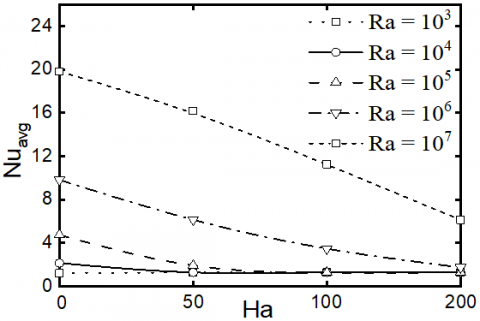Figure 8. Variation of Nuavg as a function of Ha and Ra, with φ = 5%

## 9.png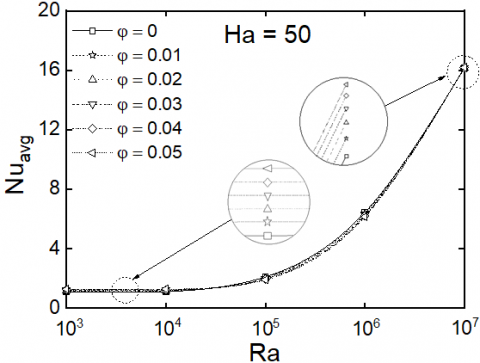Figure 9. Variation of Nuavgwith Rayleigh number and nanoparticle volume fraction with Ha = 50

Figure 9 shows the variation of Nuavg under the influence of percentage of nanoparticles for a Rayleigh number varies from 103 to 107. When Ra is low, it is correct that since the flux is mainly conduction, the heat transfer remains unchanged.

For higher Ra values, the flow has become by convection and the rate of heat transfer is improved. Figure 9 also shows that, for all Rayleigh numbers, the Nuavg increases with increasing nanoparticles volume fraction. This result is explained by the increased thermal conductivity of nanofluid.

5. Conclusion

In this study, we used a numerical approach to the finite volume method to solve conservation equations and the SIMPLEC algorithm to investigate the influence of the presence of a magnetic field in free convection in a cubic space full of nanofluids.

The results were presented for Ra (103…107), Ha (0…200) and φ (0…0.05) in form of isotherms, velocity profiles, streamlines, and average Nusselt number. The main conclusions that can be drawn are as follows.

• The increase in Ra intensifies the circulation of convective flows and leads to an acceleration of heat transfer by natural convection.
• At low value of Rayleigh number, where conduction is dominant, the Nuavg remains unchanged whatever the value of Ha.
• In the case of strong magnetic fields, a Ra = 105, convection is completely replaced by conduction, and the role of nanoparticles in improving the average Nusselt number is negligible.
• For higher Rayleigh values, where the convective flow cycle is strengthened, the magnetic field will cause the heat transfer rate to decrease.
Nomenclature
 B0 magnetic field strength, T $C_{p}$ specific heat, $\mathrm{J} \mathrm{kg}^{-1} \mathrm{~K}^{-1}$ g gravitational acceleration, $\mathrm{m} \cdot \mathrm{s}^{-2}$ h local heat transfer coefficient, $\mathrm{W} \mathrm{m}^{-2} \mathrm{~K}^{-1}$ H width of the enclosure, m Ha Hartmann number k thermal conductivity, $\mathrm{W} \mathrm{m}^{-1} \mathrm{~K}^{-1}$ Nu Nusselt number P Pressure, Pa Pr Prandtl number T dimensional temperature, °C u, v, w dimensional velocity components, $\mathrm{m} \mathrm{s}^{-1}$ U, V, W dimensionless velocity components x, y, z dimensional coordinates, m X, Y, Z dimensionless coordinates, $\mathrm{X}=\mathrm{x} / \mathrm{H}, \mathrm{Y}=\mathrm{y} / \mathrm{H}, \mathrm{Z}=\mathrm{z} / \mathrm{H}$ Greek symbols $\alpha$ thermal diffusivity, $m^{2} s^{-1}$ $\beta$ thermal expansion coefficient, $K^{-1}$ $\mu$ dynamic viscosity, $P a . s$ $v$ kinematic viscosity, $m^{2} s^{-1}$ $\theta$ dimensionless temperature, $\theta=\left(\mathrm{T}-\mathrm{T}_{\mathrm{c}}\right) /\left(\mathrm{T}_{\mathrm{b}}-\mathrm{T}_{\mathrm{c}}\right)$ $\rho$ density, $k g m^{-3}$ $\sigma$ electrical conductivity, $\Omega^{-1} m^{-1}$ $\varphi$ solid volume fraction Subscripts avg average c cold f base fluid h hot nf nanofluid p particle
References

 Choi, S.U.S., Eastman, J.A. (1995). Enhancing thermal conductivity of ﬂuids with nanoparticles. Dev Appl Non Newtonian Flows, 66: 99-106.

 Yang, Y., Zhang, Z.G., Grulke, E.A., Anderson, W.B., Wu, G. (2005). Heat transfer properties of nanoparticle-in-ﬂuid dispersions (nanoﬂuids) in laminar ﬂow. International Journal of Heat and Mass Transfer, 48(6): 1107-1116. https://doi.org/10.1016/j.ijheatmasstransfer.2004.09.038

 Khanafer, K., Vafai, K., Lightstone, M. (2003). Buoyancy-driven heat transfer enhancement in a two-dimensional enclosure utilizing nanofluid. International Journal of Heat and Mass Transfer, 46(19): 3639-3653. https://doi.org/10.1016/S0017-9310(03)00156-X

 Abu-Nada, E., Oztop, H.F. (2009). Effects of inclination angle on natural convection in enclosures filled with Cu–water nanofluid. International Journal of Heat and Fluid Flow. 30(4): 669-678. https://doi.org/10.1016/j.ijheatfluidflow.2009.02.001

 Oztop, H.F., Abu-Nada, E. (2008). Numerical study of natural convection in partially heated rectangular enclosures filled with nanofluid. International Journal of Heat and Fluid Flow, 29(5): 1326-1336. https://doi.org/10.1016/j.ijheatfluidflow.2008.04.009

 Mohebbi, R., Izadi, M., Chamkha, A.J. (2017). Heat source location and natural convection in a C-shaped enclosure saturated by a nanofluid. Physics of Fluids, 29(12): 122009. https://doi.org/10.1063/1.4993866

 Charafi, M.M., Bendaraa, A., Hasnaoui, A. (2018). Numerical modelling of natural convection in a square cavity: Effect of nanofluid volume fraction and inclination. MATEC Web Conf., 241: 01006. https://doi.org/10.1051/matecconf/201824101006

 Boudjeniba, B., Laouer, A., Laouar, S., Mezaache, E. (2019). Transition to chaotic natural convection of Cu-water nanofluid in an inclined square enclosure. International Journal of Heat and Technology, 37(2): 413-422. https://doi.org/10.18280/ijht.370206

 Sheremet, M.A., Pop, I., Mahian, O. (2018). Natural convection in an inclined cavity with time-periodic temperature boundary conditions using nanofluids: Application in solar collectors. International Journal of Heat and Mass Transfer, 116: 751-761. https://doi.org/10.1016/j.ijheatmasstransfer.2017.09.070

 Boualit, A., Zeraibi, N., Chergui, T., Lebbi, M., Boutina, L., Laouar, S. (2017). Natural convection investigation in square cavity filled with nanofluid using dispersion model. International Journal of Hydrogen Energy, 42(13): 8611-8623. https://doi.org/10.1016/j.ijhydene.2016.07.132

 Albojamal, A., Vafai, K. (2017). Analysis of single phase, discrete and mixture models, in predicting nanofluid transport. International Journal of Heat and Mass Transfer, 114: 225-237. https://doi.org/10.1016/j.ijheatmasstransfer.2017.06.030

 Roy, N.C. (2018). Natural convection of nanofluids in a square enclosure with different shapes of inner geometry. Physics of Fluids, 30(11): 113605. https://doi.org/10.1063/1.5055663

 Joshi, S., Mahapatra, S., Pattamatta, A. (2017). Effect of particle shape and slip mechanism on buoyancy induced convective heat transport with nanofluids. Physics of Fluids, 29(12): 122001. https://doi.org/10.1063/1.4996824

 Ravnik, J., Skerget, L., Hribersek, M. (2010). Analysis of three-dimensional natural convection of nanofluids by BEM. Engineering Analysis with Boundary Elements, 34(12): 1018-1030. https://doi.org/10.1016/j.enganabound.2010.06.019

 El Moutaouakil, L., Boukendil, M., Zrikem, Z., Abdelbaki A. (2020). Natural convection and thermal radiation influence on nanofluids in a cubical cavity. International Journal of Heat and Technology, 38(1): 59-68. https://doi.org/10.18280/ijht.380107

 Selimefendigil, F., Öztop, H.F. (2019). Conjugate mixed convection of nanofluid in a cubic enclosure separated with a conductive plate and having an inner rotating cylinder. International Journal of Heat Mass Transfer, 139: 1000-1017. https://doi.org/10.1016/j.ijheatmasstransfer.2019.05.053

 Atashafrooz, M. (2018). Effects of Ag-water nanofluid on hydrodynamics and thermal behaviors of three-dimensional separated step flow. Alexandria Engineering Journal, 57(4): 4277-4285. https://doi.org/10.1016/j.aej.2017.07.016

 Sajjadi, H., Delouei, A.A., Sheikholeslami, M., Atashafrooz, M., Succi, S. (2019). Simulation of three dimensional MHD natural convection using double MRT Lattice Boltzmann method. Physica A: Statistical Mechanics and its Applications, 515: 474-496. https://doi.org/10.1016/j.physa.2018.09.164

 Zhou, W., Yan, Y., Xie, Y., Liu, B. (2017). Three dimensional lattice Boltzmann simulation for mixed convection of nanoﬂuids in the presence of magnetic ﬁeld. International Communications in Heat and Mass Transfer, 80: 1-9. http://doi.org/10.1016/j.icheatmasstransfer.2016.11.012

 Al-Sayegh, R. (2018). Influence of external magnetic field inclination on three dimensional buoyancy-driven convection in an open trapezoidal cavity filled with CNT-water nanofluid. International Journal of Mechanical Sciences, 148: 756-765. https://doi.org/10.1016/j.ijmecsci.2018.09.032

 Al-Rashed, A.A.A.A., Kalidasan, K., Kolsi, L., Aydi, A., Malekshah, E.H., Hussein, A.K., Rajesh Kanna, P. (2018). Three-dimensional investigation of the effects of external magnetic field inclination on laminar natural convection heat transfer in CNT-water nanofluid filled cavity. Journal of Molecular Liquids, 252: 454-468. https://doi.org/10.1016/j.molliq.2018.01.006

 Bejan, A. (2013). Convection Heat Transfer. John Wiley & Sons, Inc., Hoboken, New Jersey, USA.

 Abu-Nada, E., Oztop, H. (2009). Effect of inclination angle on natural convection in enclosure filled with Cu-water nanofluids. International Journal of Heat and Fluid Flow, 30(4): 669-678. https://doi.org/10.1016/j.ijheatfluidflow.2009.02.001

 Patankar, S.V. (1980). Numerical Heat Transfer and Fluid Flow. Hemisphere Publishing Corporation, Taylor and Francis Group, New York.

 Van Doormaal J.P., Raithby G.D. (1984). Enhancements of the SIMPLE method for predicting incompressible fluid flows. Numerical Heat Transfer, 7(2): 147-163. https://doi.org/10.1080/01495728408961817